# Solving Equations Involving Radical Expressions

8. Mar 2021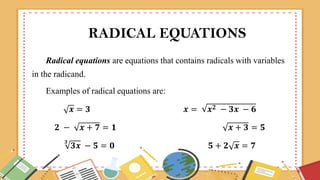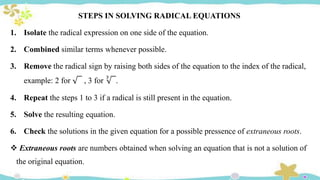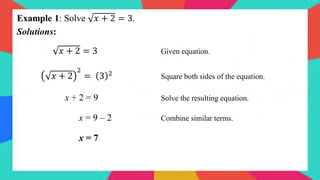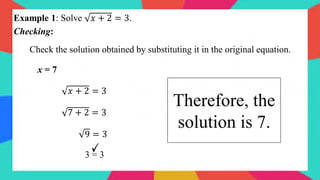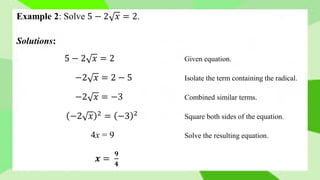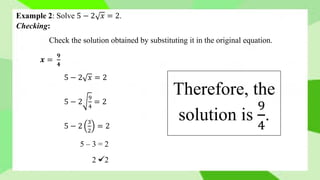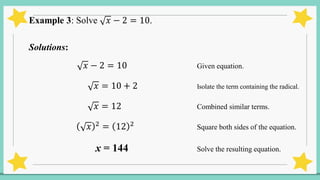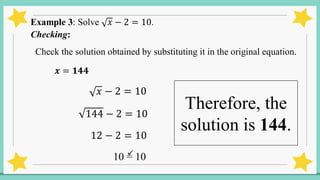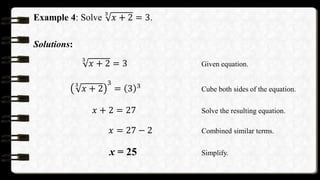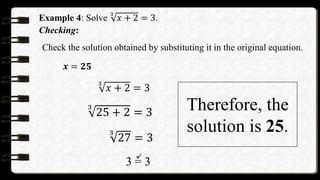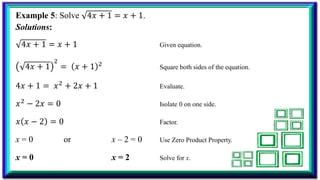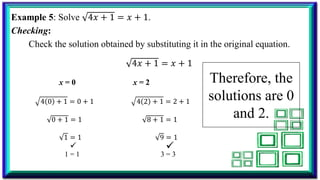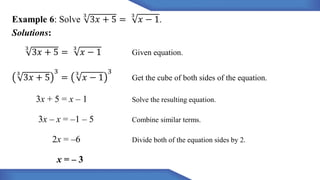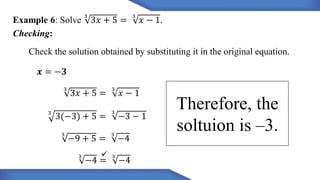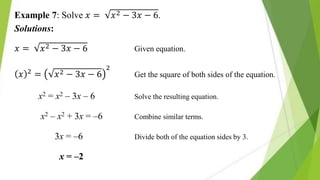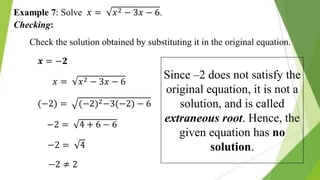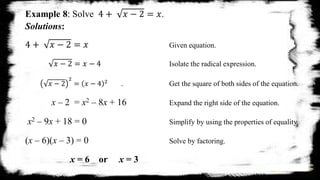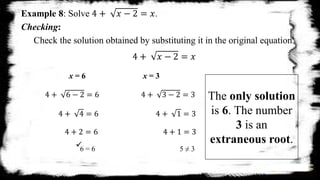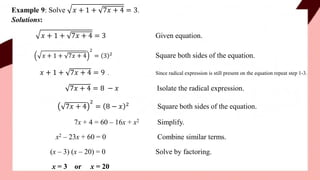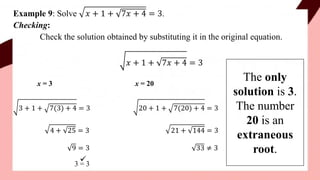1 von 22

### Solving Equations Involving Radical Expressions

• 1. MATHEMATICS 9 SOLVING EQUATIONS INVOLVING RADICAL EXPRESSIONS
• 2. RADICAL EQUATIONS Radical equations are equations that contains radicals with variables in the radicand. Examples of radical equations are: 𝒙 = 𝟑 𝒙 = 𝒙𝟐 − 𝟑𝒙 − 𝟔 𝟐 − 𝒙 + 𝟕 = 𝟏 𝒙 + 𝟑 = 𝟓 𝟑 𝟑𝒙 − 𝟓 = 𝟎 𝟓 + 𝟐 𝒙 = 𝟕
• 3. STEPS IN SOLVING RADICAL EQUATIONS 1. Isolate the radical expression on one side of the equation. 2. Combined similar terms whenever possible. 3. Remove the radical sign by raising both sides of the equation to the index of the radical, example: 2 for 1 , 3 for 3 2. 4. Repeat the steps 1 to 3 if a radical is still present in the equation. 5. Solve the resulting equation. 6. Check the solutions in the given equation for a possible pressence of extraneous roots.  Extraneous roots are numbers obtained when solving an equation that is not a solution of the original equation.
• 5. Example 1: Solve 𝑥 + 2 = 3. Solutions: 𝑥 + 2 = 3 Given equation. 𝑥 + 2 2 = 3 2 Square both sides of the equation. x + 2 = 9 Solve the resulting equation. x = 9 – 2 Combine similar terms. x = 7
• 6. Example 1: Solve 𝑥 + 2 = 3. Checking: Check the solution obtained by substituting it in the original equation. x = 7 𝑥 + 2 = 3 7 + 2 = 3 9 = 3 3 = 3 Therefore, the solution is 7.
• 7. Example 2: Solve 5 − 2 𝑥 = 2. Solutions: 5 − 2 𝑥 = 2 Given equation. −2 𝑥 = 2 − 5 Isolate the term containing the radical. −2 𝑥 = −3 Combined similar terms. −2 𝑥 2 = −3 2 Square both sides of the equation. 4x = 9 Solve the resulting equation. 𝒙 = 𝟗 𝟒
• 8. Example 2: Solve 5 − 2 𝑥 = 2. Checking: Check the solution obtained by substituting it in the original equation. 𝒙 = 𝟗 𝟒 5 − 2 𝑥 = 2 5 − 2 9 4 = 2 5 − 2 3 2 = 2 5 – 3 = 2 2 = 2 Therefore, the solution is 9 4 .
• 9. Example 3: Solve 𝑥 − 2 = 10. Solutions: 𝑥 − 2 = 10 Given equation. 𝑥 = 10 + 2 Isolate the term containing the radical. 𝑥 = 12 Combined similar terms. 𝑥 2 = 12 2 Square both sides of the equation. x = 144 Solve the resulting equation.
• 10. Example 3: Solve 𝑥 − 2 = 10. Checking: Check the solution obtained by substituting it in the original equation. 𝒙 = 𝟏𝟒𝟒 𝑥 − 2 = 10 144 − 2 = 10 12 − 2 = 10 10 = 10 Therefore, the solution is 144.
• 11. Example 4: Solve 3 𝑥 + 2 = 3. Solutions: 3 𝑥 + 2 = 3 Given equation. 3 𝑥 + 2 3 = 3 3 Cube both sides of the equation. 𝑥 + 2 = 27 Solve the resulting equation. 𝑥 = 27 − 2 Combined similar terms. x = 25 Simplify.
• 12. Example 4: Solve 3 𝑥 + 2 = 3. Checking: Check the solution obtained by substituting it in the original equation. 𝒙 = 𝟐𝟓 3 𝑥 + 2 = 3 3 25 + 2 = 3 3 27 = 3 3 = 3 Therefore, the solution is 25.
• 13. Example 5: Solve 4𝑥 + 1 = 𝑥 + 1. Solutions: 4𝑥 + 1 = 𝑥 + 1 Given equation. 4𝑥 + 1 2 = 𝑥 + 1 2 Square both sides of the equation. 4𝑥 + 1 = 𝑥2 + 2𝑥 + 1 Evaluate. 𝑥2 − 2𝑥 = 0 Isolate 0 on one side. 𝑥 𝑥 − 2 = 0 Factor. x = 0 or x – 2 = 0 Use Zero Product Property. x = 0 x = 2 Solve for x.
• 14. Example 5: Solve 4𝑥 + 1 = 𝑥 + 1. Checking: Check the solution obtained by substituting it in the original equation. 4𝑥 + 1 = 𝑥 + 1 x = 0 x = 2 4 0 + 1 = 0 + 1 4 2 + 1 = 2 + 1 0 + 1 = 1 8 + 1 = 1 1 = 1 9 = 1 1 = 1 3 = 3 Therefore, the solutions are 0 and 2.
• 15. Example 6: Solve 3 3𝑥 + 5 = 3 𝑥 − 1. Solutions: 3 3𝑥 + 5 = 3 𝑥 − 1 Given equation. 3 3𝑥 + 5 3 = 3 𝑥 − 1 3 Get the cube of both sides of the equation. 3x + 5 = x – 1 Solve the resulting equation. 3x – x = –1 – 5 Combine similar terms. 2x = –6 Divide both of the equation sides by 2. x = – 3
• 16. Example 6: Solve 3 3𝑥 + 5 = 3 𝑥 − 1. Checking: Check the solution obtained by substituting it in the original equation. 𝒙 = −𝟑 3 3𝑥 + 5 = 3 𝑥 − 1 3 3(−3) + 5 = 3 −3 − 1 3 −9 + 5 = 3 −4 3 −4 = 3 −4 Therefore, the soltuion is –3.
• 17. Example 7: Solve 𝑥 = 𝑥2 − 3𝑥 − 6. Solutions: 𝑥 = 𝑥2 − 3𝑥 − 6 Given equation. 𝑥 2 = 𝑥2 − 3𝑥 − 6 2 Get the square of both sides of the equation. x2 = x2 – 3x – 6 Solve the resulting equation. x2 – x2 + 3x = –6 Combine similar terms. 3x = –6 Divide both of the equation sides by 3. x = –2
• 18. Example 7: Solve 𝑥 = 𝑥2 − 3𝑥 − 6. Checking: Check the solution obtained by substituting it in the original equation. 𝒙 = −𝟐 𝑥 = 𝑥2 − 3𝑥 − 6 (−2) = (−2)2−3(−2) − 6 −2 = 4 + 6 − 6 −2 = 4 −2 ≠ 2 Since –2 does not satisfy the original equation, it is not a solution, and is called extraneous root. Hence, the given equation has no solution.
• 19. Example 8: Solve 4 + 𝑥 − 2 = 𝑥. Solutions: 4 + 𝑥 − 2 = 𝑥 Given equation. 𝑥 − 2 = 𝑥 − 4 Isolate the radical expression. 𝑥 − 2 2 = 𝑥 − 4 2 . Get the square of both sides of the equation. x – 2 = x2 – 8x + 16 Expand the right side of the equation. x2 – 9x + 18 = 0 Simplify by using the properties of equality. (x – 6)(x – 3) = 0 Solve by factoring. x = 6 or x = 3
• 20. Example 8: Solve 4 + 𝑥 − 2 = 𝑥. Checking: Check the solution obtained by substituting it in the original equation. 4 + 𝑥 − 2 = 𝑥 x = 6 x = 3 4 + 6 − 2 = 6 4 + 3 − 2 = 3 4 + 4 = 6 4 + 1 = 3 4 + 2 = 6 4 + 1 = 3 6 = 6 5 ≠ 3 The only solution is 6. The number 3 is an extraneous root.
• 21. Example 9: Solve 𝑥 + 1 + 7𝑥 + 4 = 3. Solutions: 𝑥 + 1 + 7𝑥 + 4 = 3 Given equation. 𝑥 + 1 + 7𝑥 + 4 2 = 3 2 Square both sides of the equation. 𝑥 + 1 + 7𝑥 + 4 = 9 . Since radical expression is still present on the equation repeat step 1-3. 7𝑥 + 4 = 8 − 𝑥 Isolate the radical expression. 7𝑥 + 4 2 = 8 − 𝑥 2 Square both sides of the equation. 7x + 4 = 60 – 16x + x2 Simplify. x2 – 23x + 60 = 0 Combine similar terms. (x – 3) (x – 20) = 0 Solve by factoring. x = 3 or x = 20
• 22. Example 9: Solve 𝑥 + 1 + 7𝑥 + 4 = 3. Checking: Check the solution obtained by substituting it in the original equation. 𝑥 + 1 + 7𝑥 + 4 = 3 x = 3 x = 20 3 + 1 + 7(3) + 4 = 3 20 + 1 + 7(20) + 4 = 3 4 + 25 = 3 21 + 144 = 3 9 = 3 33 ≠ 3 3 = 3 The only solution is 3. The number 20 is an extraneous root.